# 3 SUMIF with OR function Formulas in Excel

We know how to sum if two criteria match using SUMIFS function. But how do we sum if either of two criteria match. In other words, how do we sum if this or that. In that case conventional SUMIF formula will not work. In this article we will learn how to sum if any of the given conditions are matched. There are several methods. Let’s learn them by one example.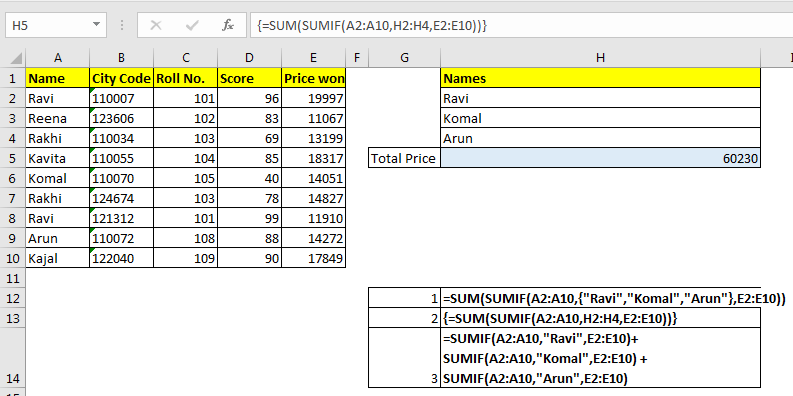SUMIF From Same Criteria Range
Here we have data of price won by some students.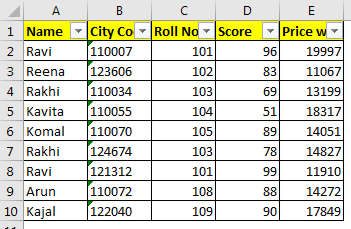Example 1: Sum Price If Name is Ravi or Reena
Here we have two conditions for summing up data. In other words, we need to sum total amount won by Ravi and Reena.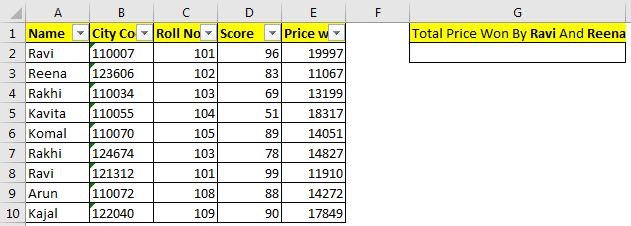Solution 1: Using SUM and SUMIF Function
The generic formula is:

=SUM(SUMIF(criteria_range,{criteria1,criteria2,...},sum_range))

We will use simple SUMIF function here. The only difference will be criterias being put into curly braces. This formula will encapsulated into SUM function. Here’s how.

For this example, the formula will be:

=SUM(SUMIF(A2:A10,{"Ravi","Reena"},E2:E10))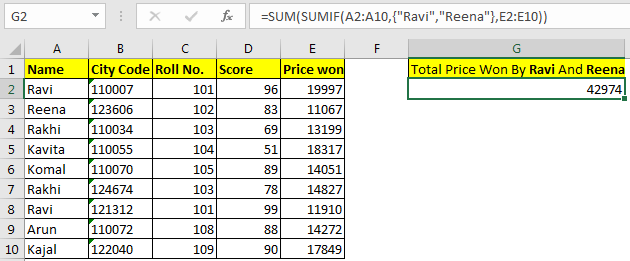How it works?
This formula works as SUM with OR logic. Let’s understand how.
Starting from inside, let’s understand this SUMIF(A2:A10,{"Ravi","Reena"},E2:E10) part. It is simple SUMIF function. But instead of one criteria, we have provided two criteria to SUMIF in an array. The SUMIF function sums up value in sumrange (E2:E10) and returns an array for both criterias. {31907,11067} (sum of “Ravi”, Sum of “Reena”).

=SUM(SUMIF(A2:A10,{"Ravi","Reena"},E2:E10)): Now we just need to add them up. To add this array we simply appointed SUM function. SUM({31907,11067}). Finally, we get our sum as 42974.

Solution 2: Adding up two SUMIF formulas
It is an straight forward solution for obtaining a SUMIF with Or condition. We just add two SUMIF formulas.

=SUMIF(A2:A10,"Ravi",E2:E10)+SUMIF(A2:A10,"Reena",E2:E10)

But it is only feasible when you have fixed and very few number of criterias. It will be a long formula if criteria are more.
Example 2: Sum if with Or Statement From Cell References.
In above example we had fixed names, but what if we want sum total price of some names written in a range. Like the snapshot below.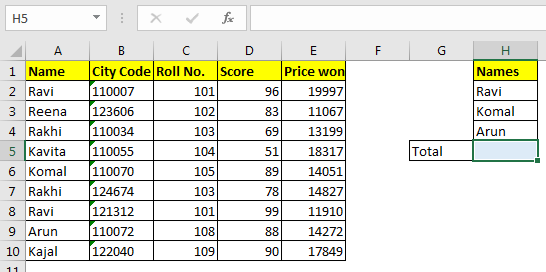Here we need to get total price won by all these three students. These names can change too. In the example above, we hardcoded the criterias, but here we need to give reference of range H2:H4.
The Generic Formula

{=SUM(SUMIF(criteria_range,criterias,sum_range))}

Note: the formula is an array formula. Entered with CTRL+SHIFT+ENTER.

The formula for our example will be:

{=SUM(SUMIF(A2:A10,H2:H4,E2:E10))}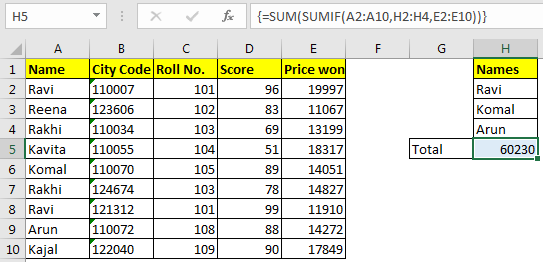How It Works

It works same as example 1 method. But in this case we have used a range instead of hardcoding the criteria. Using this method, you can give a long list for summing up with or condition. This will be dynamic too.
Important: This is an array formula and need to be entered with CTRL+SHIFT+ENTER.

In all above examples, you can use SUMIFS function instead of SUMIF. There will be no difference in results.

So yeah guys, this how you can do excel SUMIF with OR. Let me know if you have any specific query regarding excel sumif or any other excel function, feature or formula. The comments section is all yours. Till then you can read our best articles about function.

Related Articles:

SUMIFS using AND-OR logic in Excel

How to use the SUMIF Function in Excel

How to use the SUMIFS Function in Excel

How to Sum if cells contain specific text in Excel

How to Sum If Greater Than 0 in Excel

Get SUMIFS with dates in Excel

Popular Articles :

50 Excel Shortcut to Increase Your Productivity : Get faster at your task. These 50 shortcuts will make you work even faster on Excel.

How to use the VLOOKUP Function in Excel : This is one of the most used and popular functions of excel that is used to lookup value from different ranges and sheets.

How to use the COUNTIF function in Excel : Count values with conditions using this amazing function. You don't need to filter your data to count specific values. Countif function is essential to prepare your dashboard.

How to use the SUMIF Function in Excel : This is another dashboard essential function. This helps you sum up values on specific conditions.

Terms and Conditions of use

The applications/code on this site are distributed as is and without warranties or liability. In no event shall the owner of the copyrights, or the authors of the applications/code be liable for any loss of profit, any problems or any damage resulting from the use or evaluation of the applications/code.